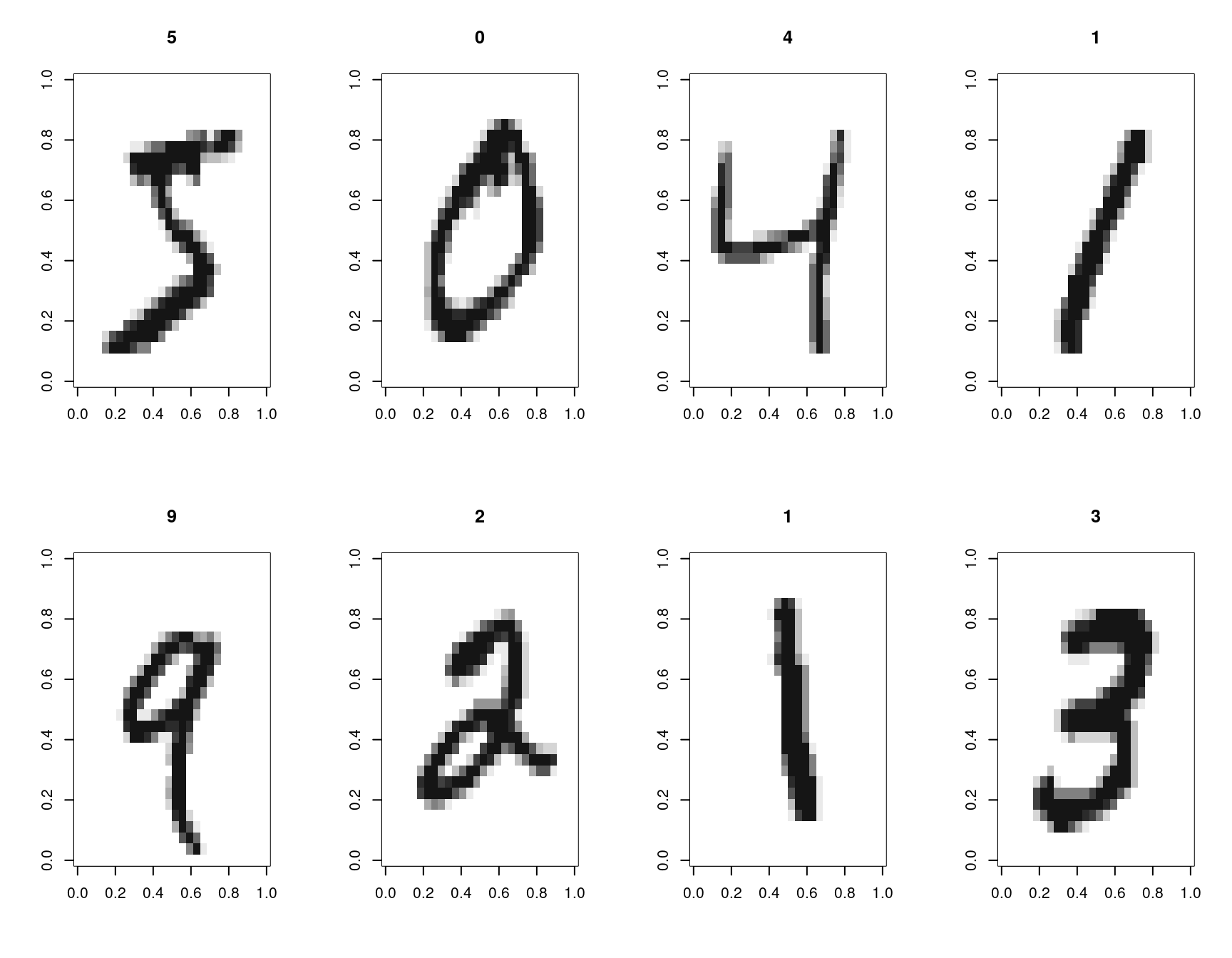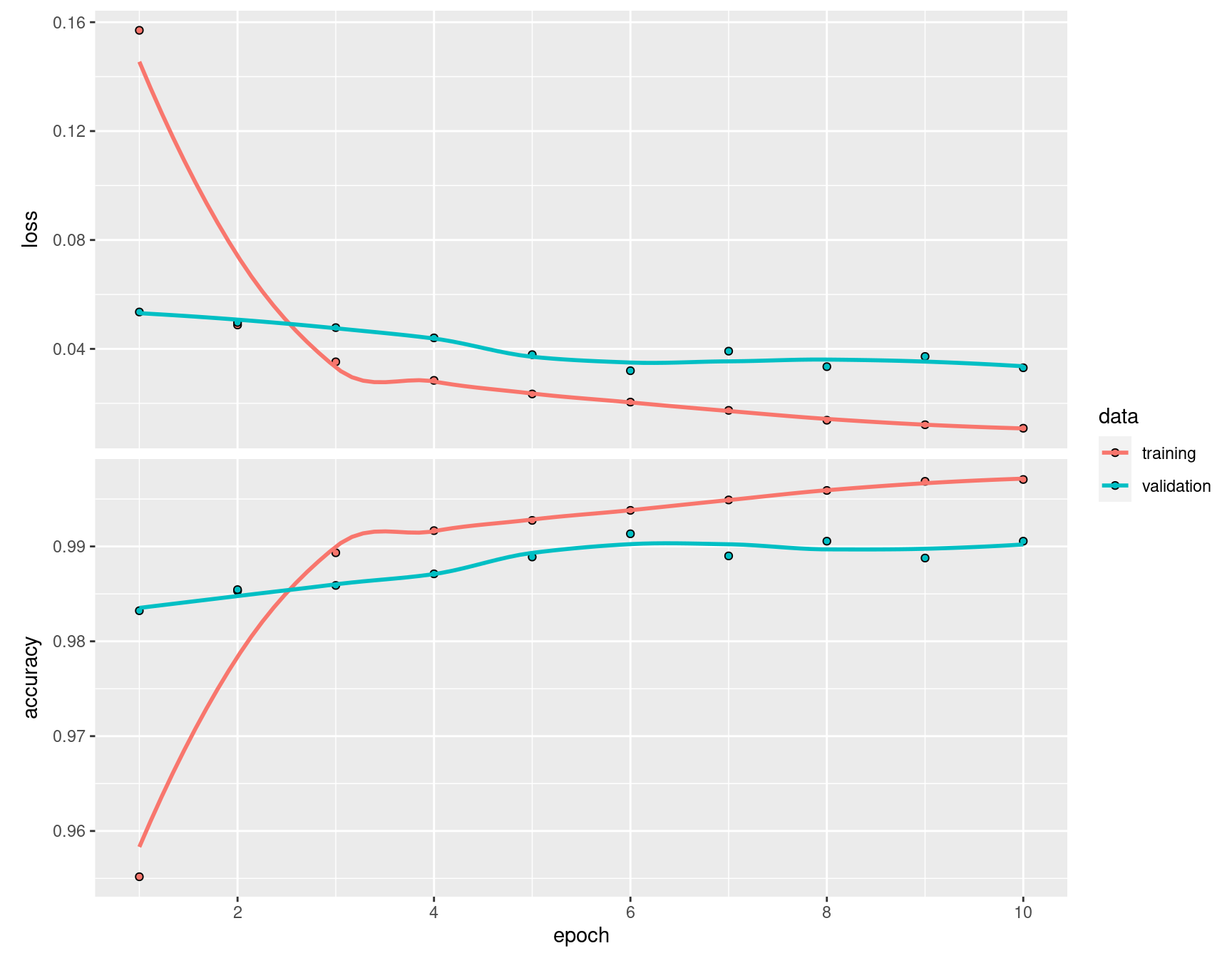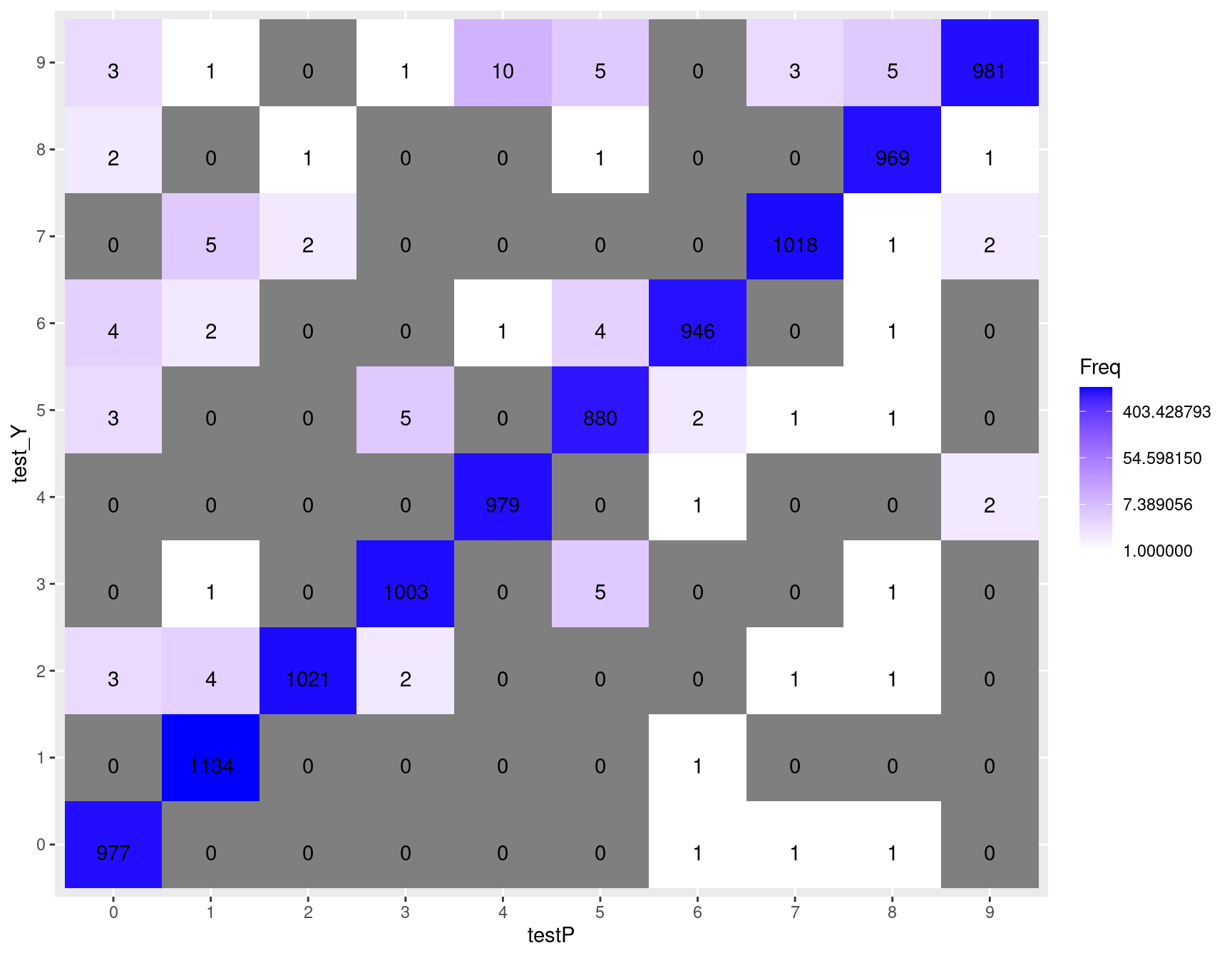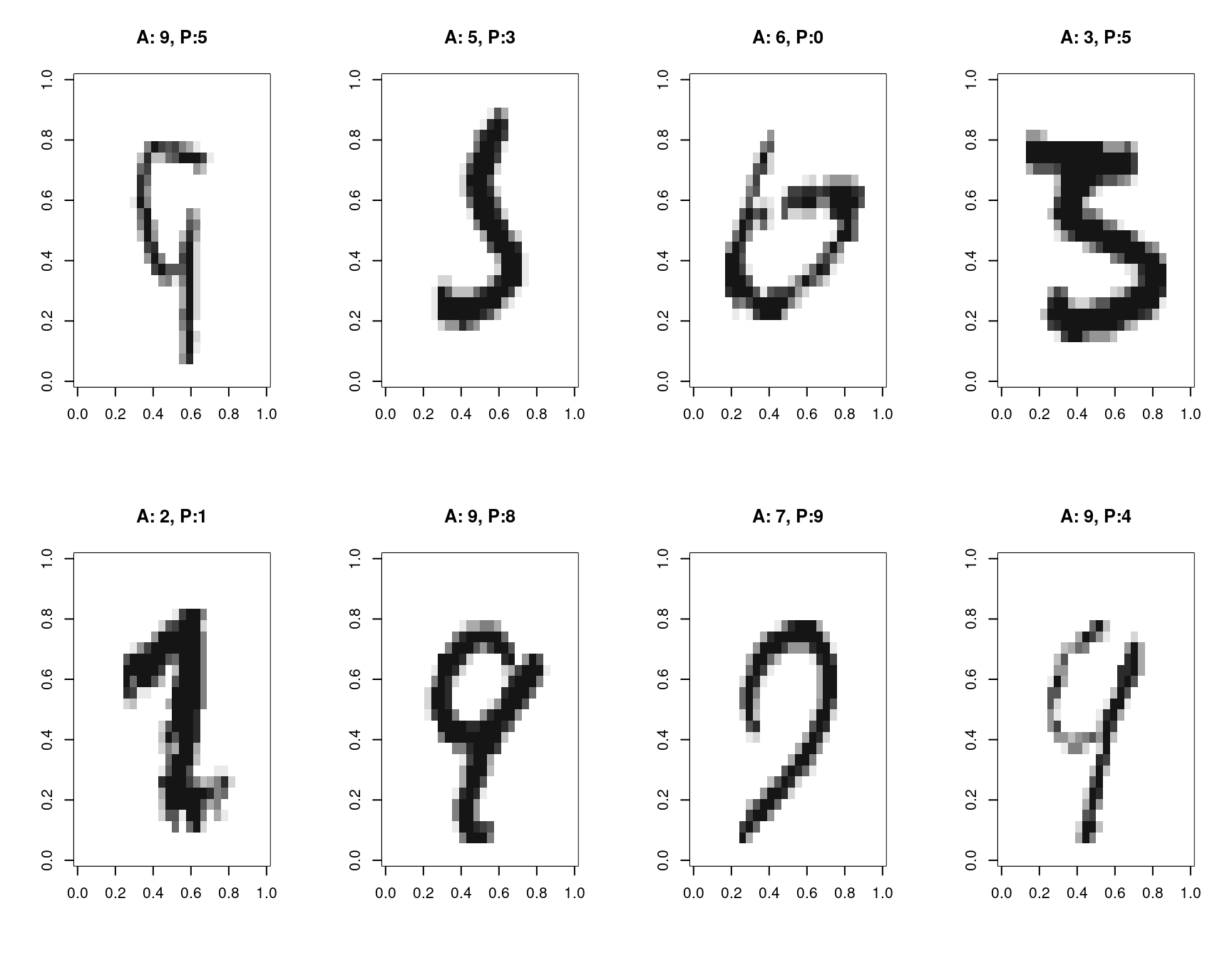# Disclaimer

This notebook was created for the SAV block course “Deep Learning with Actuarial Applications in R”.

The course is based on the publications on the following website: https://www.actuarialdatascience.org/

Author: Daniel Meier

# Applying Convolutional Neural Networks for classification of handwritten digits

## Abstract

The MNIST dataset, i.e. images of handwriten digits to be recognized, is a standard dataset to illustrate the strengths of (deep) convolutional neural networks (CNNs). In this notebook we construct a 7-layer CNN (not counting the batch normalizations separately) with 3 pairs of a convolutional layer followed by max pooling, and a final fully connected layer with 10 outputs for each of the 10 digits.

## Introduction

The MNIST dataset consists of 70’000 monochrome pictures of pixel size 28 x 28 and is already split into a training set of 60’000 pictures and test set of 10’000 pictures.

The constructed CNN is a 7-layer network comprising

• a convolutional 2D layer: 10 filters of size 3 times 3 and stepsize 1 and 1,
• a max pooling layer: window size 2 times 2, stepsize 2 and 2,
• a convolutional 2D layer: 20 filters of size 3 times 3 and stepsize 1 and 1,
• a max pooling layer: window size 2 times 2, stepsize 1 and 1,
• a convolutional 2D layer: 40 filters of size 3 times 3 and stepsize 1 and 1,
• a max pooling layer: window size 2 times 2, stepsize 2 and 2,
• a fully connected layer.

We formulate the problem as a classification problem minimizing the categorical crossentropy and consider the resulting multi-class accuracy as metric.

In Section 0 we import all necessary modules and define the most relevant parameters. In Section 1 we load the MNIST dataset and plot some examples. Section 2 constructs the CNN and applies it on the MNIST dataset. Section 3 plots the accuracy history and the confusion matrix across all 10 digits, and Section 4 shows all wrongly classified images. Section 5 considers how translations, rotations, scalings affect model performance.

## 0. Import modules, definition of parameters

``````options(encoding = 'UTF-8')

library("repr")  # not needed in the Rmarkdown version, only for Jupyter notebook
library("ggplot2")
library("keras")
library("tensorflow")
library("OpenImageR")``````
``````knitr::opts_chunk\$set(fig.width = 9, fig.height = 7)
#options(repr.plot.width=4, repr.plot.height=10)``````
``````validationRatio <- 0.15
filterSize1     <- 3
numberFilters1  <- 10
filterSize2     <- 3
numberFilters2  <- 20
filterSize3     <- 3
numberFilters3  <- 40
numberEpochs    <- 10

dataRoot <- "../../data"``````

``````load_image_file <- function(filename) {
ret = list()
f = file(filename, 'rb')
readBin(f, 'integer', n = 1, size = 4, endian = 'big')
n    = readBin(f, 'integer', n = 1, size = 4, endian = 'big')
nrow = readBin(f, 'integer', n = 1, size = 4, endian = 'big')
ncol = readBin(f, 'integer', n = 1, size = 4, endian = 'big')
x = readBin(f, 'integer', n = n * nrow * ncol, size = 1, signed = FALSE)
close(f)
data.frame(matrix(x, ncol = nrow * ncol, byrow = TRUE))
}

load_label_file <- function(filename) {
f = file(filename, 'rb')
readBin(f, 'integer', n = 1, size = 4, endian = 'big')
n = readBin(f, 'integer', n = 1, size = 4, endian = 'big')
y = readBin(f, 'integer', n = n, size = 1, signed = FALSE)
close(f)
y
}

trainX <- load_image_file(file.path(dataRoot, "cnn2", "train-images.idx3-ubyte"))
testX  <- load_image_file(file.path(dataRoot, "cnn2", "t10k-images.idx3-ubyte"))

train_Y <- as.factor(load_label_file(file.path(dataRoot, "cnn2", "train-labels.idx1-ubyte")))
test_Y  <- as.factor(load_label_file(file.path(dataRoot, "cnn2", "t10k-labels.idx1-ubyte")))

trainX <- array_reshape(data.matrix(trainX) / 255, c(dim(trainX), 28, 28, 1))
testX <- array_reshape(data.matrix(testX) / 255, c(dim(testX), 28, 28, 1))
trainY <- to_categorical(train_Y, 10)
testY <- to_categorical(test_Y, 10)

par(mfrow = c(2, 4))
for (j in 1:8) {
image(aperm(trainX[j, 28:1, , 1], c(2, 1)), col = gray(12:1 / 12))
title(train_Y[j])
}``````## 2. Constructing and fitting the CNN

``````set.seed(0)
tf\$random\$set_seed(0)

cnn <- keras_model_sequential() %>%
layer_conv_2d(filters = numberFilters1, kernel_size = c(filterSize1, filterSize1),
strides = c(1,1), padding = 'valid', input_shape = c(28, 28, 1)) %>%
layer_batch_normalization() %>%
layer_activation('relu') %>%
layer_max_pooling_2d(pool_size = c(2,2), strides = c(2,2), padding = 'valid') %>%

layer_conv_2d(filters = numberFilters2, kernel_size = c(filterSize2, filterSize2),
strides = c(1,1), padding = 'valid') %>%
layer_batch_normalization() %>%
layer_activation('relu') %>%
layer_max_pooling_2d(pool_size = c(2,2), strides = c(1,1), padding = 'valid') %>%

layer_conv_2d(filters = numberFilters3, kernel_size = c(filterSize3, filterSize3),
strides = c(1,1), padding = 'valid') %>%
layer_batch_normalization() %>%
layer_activation('relu') %>%
layer_max_pooling_2d(pool_size = c(2,2), strides = c(2,2)) %>%

layer_flatten() %>%
layer_dense(10) %>%
layer_activation('softmax', name = 'softmax') %>%
compile(loss = loss_categorical_crossentropy, optimizer = optimizer_adadelta(), metrics = c('accuracy'))

# RSc: below took ~22 minutes with 1CPU / 8GB / 40 epochs
summary <- cnn %>% fit(
x = trainX,
y = trainY,
epochs = numberEpochs,
validation_split = validationRatio,
batch_size = 64,
verbose = 1
)
summary(cnn)``````
``````## Model: "sequential"
## ________________________________________________________________________________
## Layer (type)                        Output Shape                    Param #
## ================================================================================
## conv2d_2 (Conv2D)                   (None, 26, 26, 10)              100
## ________________________________________________________________________________
## batch_normalization_2 (BatchNormali (None, 26, 26, 10)              40
## ________________________________________________________________________________
## activation_2 (Activation)           (None, 26, 26, 10)              0
## ________________________________________________________________________________
## max_pooling2d_2 (MaxPooling2D)      (None, 13, 13, 10)              0
## ________________________________________________________________________________
## conv2d_1 (Conv2D)                   (None, 11, 11, 20)              1820
## ________________________________________________________________________________
## batch_normalization_1 (BatchNormali (None, 11, 11, 20)              80
## ________________________________________________________________________________
## activation_1 (Activation)           (None, 11, 11, 20)              0
## ________________________________________________________________________________
## max_pooling2d_1 (MaxPooling2D)      (None, 10, 10, 20)              0
## ________________________________________________________________________________
## conv2d (Conv2D)                     (None, 8, 8, 40)                7240
## ________________________________________________________________________________
## batch_normalization (BatchNormaliza (None, 8, 8, 40)                160
## ________________________________________________________________________________
## activation (Activation)             (None, 8, 8, 40)                0
## ________________________________________________________________________________
## max_pooling2d (MaxPooling2D)        (None, 4, 4, 40)                0
## ________________________________________________________________________________
## flatten (Flatten)                   (None, 640)                     0
## ________________________________________________________________________________
## dense (Dense)                       (None, 10)                      6410
## ________________________________________________________________________________
## softmax (Activation)                (None, 10)                      0
## ================================================================================
## Total params: 15,850
## Trainable params: 15,710
## Non-trainable params: 140
## ________________________________________________________________________________``````

## 3. Accuracy history and confusion matrix

Exercise: Experiment with other structures/parameters of the CNN. Make use of summary(cnn) to check the dimensions of inputs/outputs of each layer. How are the dimensions affected by strides, padding, kernel_size, number of filters?

Exercise: Change the random seeds (`set.seed(0)` and `tf\$random\$set_seed(0)`). If you keep the random seeds, are the results 100% reproducible?

Exercise: Change the relu activation functions to some other activation functions.

Exercise: The input images are gray scale images. Turn them into black-white images (only allowing values 0 and 1) and refit the model.

Exercise: Introduce some random noise in the images, e.g. by adding i.i. uniformly distributed numbers out of the interval [-r,r]. Plot r vs accuracy for some selected r.

Exercise: Set 0<r<28^2 random pixels to white and plot r vs accuracy for some selected r.

Exercise: There are several other structures/parameters to be found in the web, e.g. https://keras.rstudio.com/articles/examples/mnist_cnn.html, or https://tensorflow.rstudio.com/guide/keras/, etc. not all of them necessarily make use of convolutional layers. What are the main differences between these structures? Advantages/disadvantages? Which performs best?

``plot(summary)``
``## `geom_smooth()` using formula 'y ~ x'````print(summary)``
``````##
## Final epoch (plot to see history):
##         loss: 0.01086
##     accuracy: 0.9971
##     val_loss: 0.03306
## val_accuracy: 0.9906``````
``````#testP <- cnn %>% predict_classes(testX)  # This is deprecated in keras/tf 2.6. In our case, below is applicable instead.
testP <- cnn %>% predict(testX) %>% k_argmax()
testP <- as.array(testP)

confusion_matrix <- as.data.frame(table(testP, test_Y))

ggplot(data = confusion_matrix, aes(x = testP, y = test_Y)) +
geom_tile(aes(fill = Freq)) +
geom_text(aes(label = sprintf("%1.0f", Freq)), vjust = 1) +
scale_fill_gradient(low = "white", high = "blue", trans = "log")``````
``## Warning: Transformation introduced infinite values in discrete y-axis``## 4. Wrongly classified images

Images where the actual image differs from the predicted image (denoted as A and P) are shown in this section.

``````incorrectIdx <- which(test_Y != testP)
par(mfrow = c(2, 4))
for (j in 1:8) {
image(aperm(testX[incorrectIdx[j], 28:1, , 1], c(2,1)), col = gray(12:1 / 12))
title(paste0('A: ', test_Y[incorrectIdx[j]], ', P:', testP[incorrectIdx[j]]))
}````````print(paste(length(incorrectIdx), "incorrectly classified digits (out of 10'000 digits)"))``
``##  "92 incorrectly classified digits (out of 10'000 digits)"``

## 5. Rotations, translations, scalings

The following 3 cells (chunks) rotate, translate and scale images. Observe how the model predictions (the softmax layer) are impacted by these transformations.

``````layerModel <- keras_model(input = cnn\$input, outputs = get_layer(cnn, 'softmax')\$output)  # the softmax activation layer
img <- trainX[19, 28:1, , ]
par(mfrow = c(2, 4))
for (j in seq(0, 315, 45)) {
image(aperm(rotateImage(img, j), c(2,1)), col = gray(12:1 / 12))
title(j)
}``````